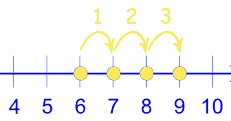Here are lots of "thinking tricks" you can use to make addition easier.

Use the ones that make sense to you!

## Count From A Number Upwards

### Example: 6 + 3Think "6 ... 7, 8, 9"

Hint: start from the larger number.

### Example: 2 + 6

2 + 6 is Harder: "2 ... 3, 4, 5, 6, 7, 8"

6 + 2 is Easier: "6 ... 7, 8"

## Jump Strategy

We can also count by 2s or 10s, or make any "jumps" we want to help us solve a calculation.

### Example: 4 + 12Think "4 ... 14 ... 15, 16"

See if any numbers add to 10. They don't have to be next to each other.

### Example: 7 + 8 + 3 + 2 + 5

7+3 is 10,

8+2 is another 10, which makes 20,

Plus 5 is 25

## Do The Tens Last

Break big numbers into Tens and Units, add the Units, then add on the Tens.### Example: 14+5

Break the "14" into Tens and Units: 10 + 4

Add the Units: 4 + 5 = 9

Now add the Tens: 10 + 9 = 19

Think "4 plus 5 is 9, plus 10 is 19"

### Another Example: 14 + 12

Break into Tens and Units: 10 + 4 + 10 + 2

Add the Units: 4 + 2 = 6

Now add on the Tens: 6 + 10 + 10 = 26

## Aim for Ten

When a number is close to ten we can "borrow" from the other number so it reaches ten.

### Example: 9 + 7

9 is only 1 away from 10

so take 1 from the 7: 9 + 1 + 6
and give it to the 9: 10 + 6 = 16

Think "9 plus 1 is 10 ... 7 less 1 is 6 ... together that is 16"

### Example: 8 + 5

8+2=10, so lets take 2 from the 5: 8 + 2 + 3
and give it to the 8: 10 + 3 = 13

We can also move backwards to ten, by making the other number bigger as needed:

### Example: 12 + 7

Reduce 12 by 2:  12 − 2 = 10
Increase 7 by 2:  7 + 2 = 9

12 + 7 = 10 + 9 = 19

## Compensation Method

"Compensation" is where you round up a number (to make adding easier) and then take away the extra after you have added.

### Example 19+16

It is easier to do 20 + 16 = 36

Then take away the extra 1 (that made 19 into 20) to get: 35

### Example 395 + 126

It is easier to do 400 + 126 = 526

Then take away the extra 5 (that made 395 into 400) to get: 521

## Double if the numbers are close, then fix

### Example: 5 + 6 is nearly two 5s, but 6 is 1 bigger than 5, so:

5 + 6 = two 5s + 1 = 10 + 1 = 11

### Example: 7 + 9 is like two 8s, but 7 is 1 smaller than 8, and 9 is 1 bigger than 8. So it is exactly two 8s because 1 smaller and 1 bigger cancel each other:

7 + 9 = "8 less 1" + "8 add 1" = two 8s = 16